# Traceroute - Cisco, Linux, and Windows versions

In my today post I’d like analyze different version of traceroute on three platforms. You need to know exactly what protocols/types are used when you would like to permit them on your firewall.

1) Cisco version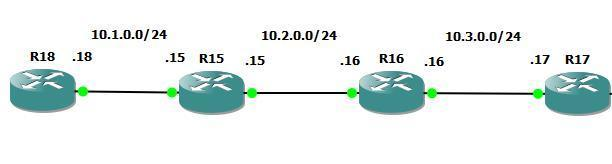I’m going now traceroute from R17 to R18: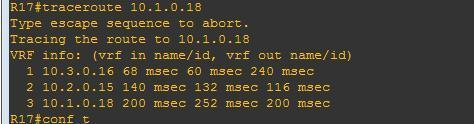This is what I captured on R17 interface: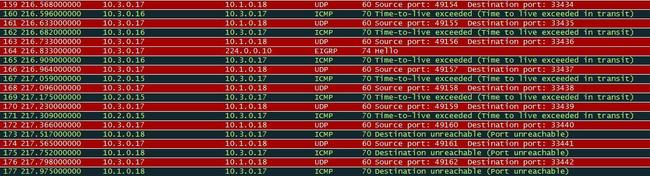Let me explain what we see:

R17 sends udp packet with ttl=1 to discover device in one hop distance. R16 decrements ttl by 1 and sees that ttl=0 and sends icmp packet ‘time exceeded):

``````-> udp - dst port: 33434, ttl=1
<- icmp - time exceeded (due to ttl=0) - type 11, code 0 - ttl=255

-> udp - dst port: 33435, ttl=1
<- icmp - time exceeded (due to ttl=0) - type 11, code 0 - ttl=255

-> udp - dst port: 33436, ttl=1
<- icmp - time exceeded (due to ttl=0) - type 11, code 0 - ttl=255
``````

After three repeats R17 increases ttl by 1 and sends next three packets. R15 receives them, decreases ttl by 1 and due to ttl=0 R15 sends back icmp 'time exceeeded’:

``````-> udp - dst port: 33437, ttl=2
<- icmp - time exceeded - type 11, code 0 - ttl=254

-> udp - dst port: 33438, ttl=2
<- icmp - time exceeded - type 11, code 0 - ttl=254

-> udp - dst port: 33439, ttl=2
<- icmp - time exceeded - type 11, code 0 - ttl=254
``````

After next three packets R17 increases ttl by 1 to ttl=3. These three packets reach R18 and due to invalid udp port (range 33434-33464) R18 sends icmp message: port unreachable (type:3, code:3):

``````-> udp - dst port: 33440, ttl=3
<- icmp - port unreachable - type 3, code 3 - ttl=253

-> udp - dst port: 33441, ttl=3
<- icmp - port unreachable - type 3, code 3 - ttl=253

-> udp - dst port: 33442, ttl=3
<- icmp - port unreachable - type 3, code 3 - ttl=253
``````

On firewall we need to open following (returning) packets: icmp time-exceeded (type 11, code 0) and port unreachable (type 3, code 3).

2) Linux version

I added Linux machine to my network: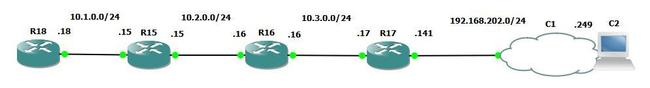and I traceroute from Linux to r18: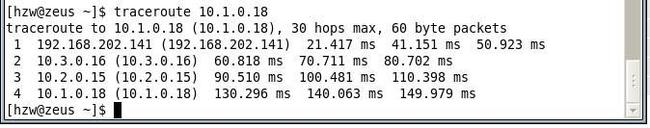Below you can see what I captured: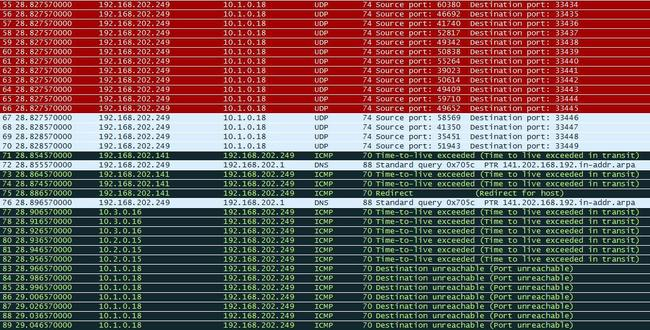Let’s analyze what we see:

Linux sends udp packet with ttl=1 to discover device in one hop distance. R17 decrements ttl by 1 and sees that ttl=0 and sends icmp packet ‘time exceeded):

``````-> udp - dst port: 33434, ttl=1
<- icmp - time exceeded (due to ttl=0) - type 11, code 0 - ttl=255

-> udp - dst port: 33435, ttl=1
<- icmp - time exceeded (due to ttl=0) - type 11, code 0 - ttl=255

-> udp - dst port: 33436, ttl=1
<- icmp - time exceeded (due to ttl=0) - type 11, code 0 - ttl=255
``````

After three repeats Linux increases ttl by 1 and sends next three packets. R16 receives them, decreases ttl by 1 and due to ttl=0 R16 sends back icmp 'time exceeeded’:

``````-> udp - dst port: 33437, ttl=2
<- icmp - time exceeded - type 11, code 0 - ttl=254

-> udp - dst port: 33438, ttl=2
<- icmp - time exceeded - type 11, code 0 - ttl=254

-> udp - dst port: 33439, ttl=2
<- icmp - time exceeded - type 11, code 0 - ttl=254
``````

After three repeats Linux increases ttl by 1 (to 3) and sends next three packets. R15 receives them, decreases ttl by 1 and due to ttl=0 R15 sends back icmp ‘time exceeeded’:

``````-> udp - dst port: 33440, ttl=3
<- icmp - time exceeded - type 11, code 0 - ttl=253

-> udp - dst port: 33441, ttl=3
<- icmp - time exceeded - type 11, code 0 - ttl=253

-> udp - dst port: 33442, ttl=3
<- icmp - time exceeded - type 11, code 0 - ttl=253
``````

In the last step Linux sends 7 udp packets with ttl=4 (3 packets), ttl=5 (3 packets) and ttl=6 (1 packet). Every packet generates responds: icmp port-unreachable.

``````-> udp - dst port: 33443, ttl=4
<- icmp - port unreachable - type 3, code 3 - ttl=252

-> udp - dst port: 33444, ttl=4
<- icmp - port unreachable - type 3, code 3 - ttl=252

-> udp - dst port: 33445, ttl=4
<- icmp - port unreachable - type 3, code 3 - ttl=252
``````
``````-> udp - dst port: 33446, ttl=5
<- icmp - port unreachable - type 3, code 3 - ttl=252

-> udp - dst port: 33447, ttl=5
<- icmp - port unreachable - type 3, code 3 - ttl=252

-> udp - dst port: 33448, ttl=5
<- icmp - port unreachable - type 3, code 3 - ttl=252
``````
``````-> udp - dst port: 33449, ttl=6
<- icmp - port unreachable - type 3, code 3 - ttl=252
``````

As you see more or less the variation is similar to the Cisco one. I can’t find any information why Linux (2.6.32) needs to send last 7 packets instead of 3.

3) Windows version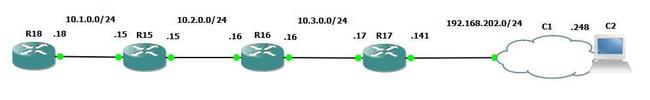For this version I use the same scenario as for linux.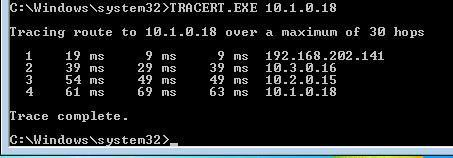The Windows version uses only icmp packets (no udp). Below you can see what I captured: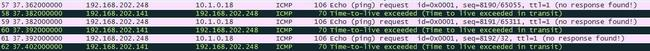``````-> icmp - echo request, ttl=1
<- icmp - time exceeded (due to ttl=0) - type 11, code 0 - ttl=255

-> icmp - echo request, ttl=1
<- icmp - time exceeded (due to ttl=0) - type 11, code 0 - ttl=255

-> icmp - echo request, ttl=1
<- icmp - time exceeded (due to ttl=0) - type 11, code 0 - ttl=255
``````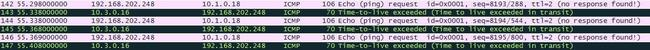``````-> icmp - echo request, ttl=2
<- icmp - time exceeded (due to ttl=0) - type 11, code 0 - ttl=254

-> icmp - echo request, ttl=2
<- icmp - time exceeded (due to ttl=0) - type 11, code 0 - ttl=254

-> icmp - echo request, ttl=2
<- icmp - time exceeded (due to ttl=0) - type 11, code 0 - ttl=254
``````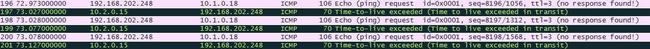``````-> icmp - echo request, ttl=3
<- icmp - time exceeded (due to ttl=0) - type 11, code 0 - ttl=253

-> icmp - echo request, ttl=3
<- icmp - time exceeded (due to ttl=0) - type 11, code 0 - ttl=253

-> icmp - echo request, ttl=3
<- icmp - time exceeded (due to ttl=0) - type 11, code 0 - ttl=253
``````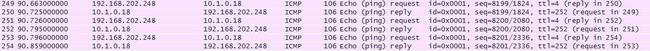``````-> icmp - echo request, ttl=4
<- icmp - echo reply - type 11, code 0 - ttl=252

-> icmp - echo request, ttl=4
<- icmp - echo reply - type 11, code 0 - ttl=252

-> icmp - echo request, ttl=4
<- icmp - echo reply - type 11, code 0 - ttl=252
``````

For Windows version, on a firewall we need to open: icmp time exceeded and echo-reply (in case we don’t inspect icmp traffic).